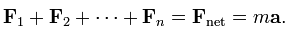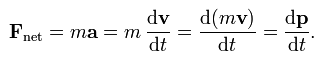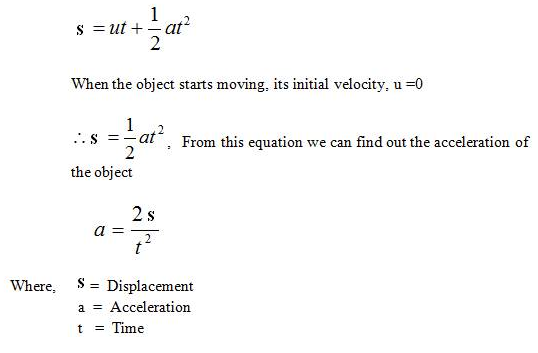Verification of Newton's Second Law

# Can you define Newton’s Second Law of Motion?

Newton's Second Law  of motion states that the rate of change of momentum of an object is proportional to the applied unbalanced force in the direction of the force.
ie.,      F=ma

Where F is the force applied, m is the mass of the body, and a, the acceleration produced.

# What will happen if a body is subject to multiple forces at the same time?

If a body is subjected to multiple forces at the same time, then the acceleration produced is proportional to the vector sum (that is, the net force) of all the individual forces.The Second Law can also be shown to relate the net force and the momentum p of the body:Therefore, Newton's Second Law also states that the net force acting on a body is equal to the rate of change of momentum of the body.# How does momentum affect the net force?

The First Law of Motion indicates that when an unbalanced external force acts on an object, its velocity changes, ie., the object is accelerated. Now, let’s  study how the acceleration of an object depends on the force applied. Consider the example. A car at rest does not require much attention when parked along a road side. But the moving car, even at a low speed, may kill a person standing in its path. A small mass, such as a bullet may kill a person when fired from a gun. From these examples it is clear that the impact produced by the objects depends on their mass and velocity. When we combine the mass and velocity of an object, we get another quantity called momentum, which was introduced by Newton.

# Let’s define Momentum:

Momentum has both direction and magnitude. Its direction is the same as that of velocity. When an unbalanced force is applied on an object, it changes the velocity of an object, which in turn changes its momentum. So, it is concluded that the force necessary to change the momentum of an object depends on the time rate at which the momentum is changed.

The acceleration of a moving object can be calculated by:## LEARNING OUTCOME:

1. Mass
2. Acceleration
3. Force
4. Momentum
5. Newton's Second Law of Motion

### Let’s understand the concept behind  Newton’s Second Law by a simple experiment.

Cite in Scientific Research:

Nedungadi P., Raman R. & McGregor M. (2013, October). Enhanced STEM learning with Online Labs: Empirical study comparing physical labs, tablets and desktops. In Frontiers in Education Conference, 2013 IEEE (pp. 1585-1590). IEEE.

Cite this Simulator: